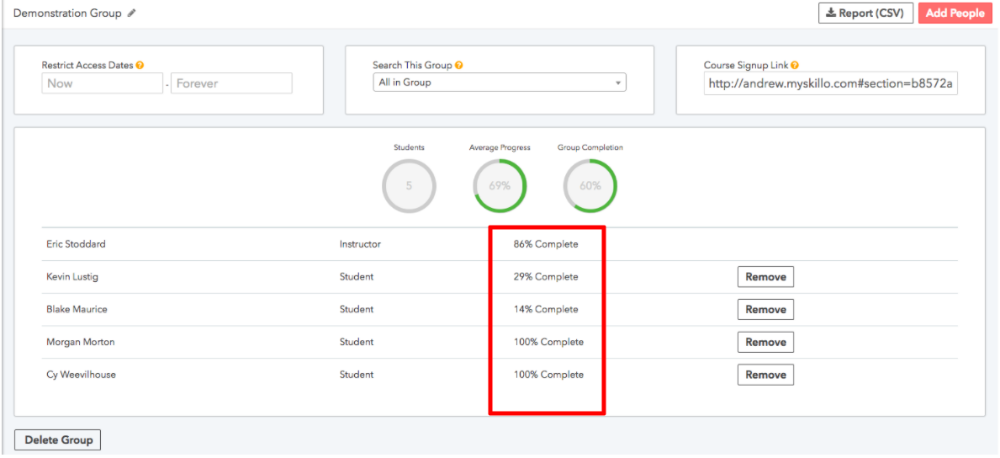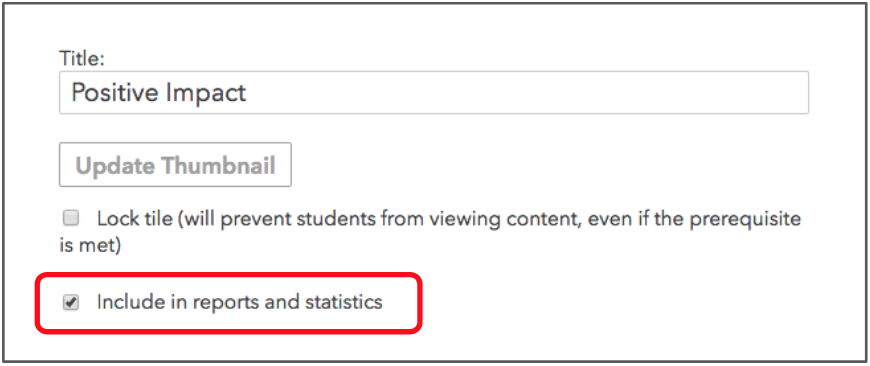# How is the completion percentage calculated?

The completion percentage is calculated by dividing the number of tiles the student has completed by the total number of tiles in the course.Note: If the "Include in reports and statistics" checkbox is not checked, that tile will not be included when calculating a student's completion percentage.